Integrating surfaces and solids of revolution with ���wheels���
P(p) is the parameter range of P.Sliders to set roots of polynomial f(p):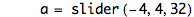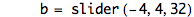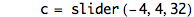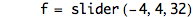Scaling factor for polynomial f(p):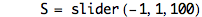f(p) is the function to be revolved around axis.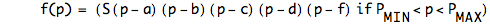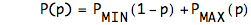Area of sector (of a circle) of angle a :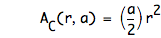Area of an annular (flat ring) sector with outer radius r , innner radius s, and angle a:Volume of a disk-sector of radius r, angle a, and thickness T: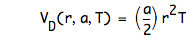Volume of the sector of a wheel of square cross-section with outer radius r , innner radius s, angle a, and thickness T :The edges of a rectangle with left at l, bottom at b, width w and height h.
p is the drawing parameter.Filling in the interior of the rectangle:
u is the horizontal and v is the vertical parameter.Plots
Dots at minimum and maximum x-values: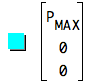Plot the curve on the xy plane: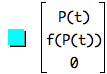Rotation around the x-axis by the angle a: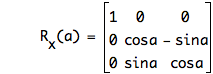Rotate the curve: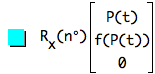The interiors of the rectangles, at start and rotated:
function(I,K*W,0,W,(K*W)^2,u,v),in(k,set(4,5,6,7,8,9,10))
function(R_x,n*degree)*function(I,K*W,0,W,(K*W)^2,u,v),in(k,set(4,5,6,7,8,9,10))

The width of the wheels: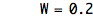The edges of the rectangles, at start: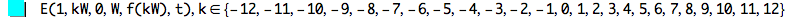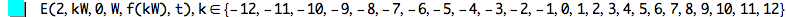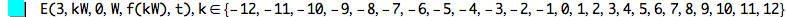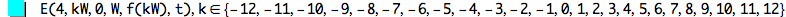The edges of the rectangles, rotated: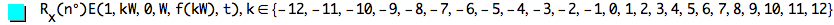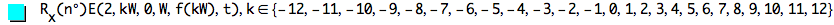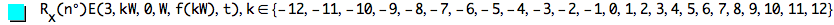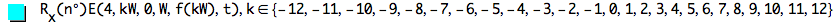The surfaces swept out by the edges: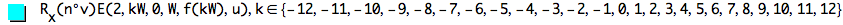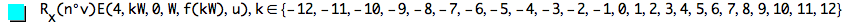This file was created by Graphing Calculator 3.5.
Visit Pacific Tech to download the helper application to view and edit these equations live.